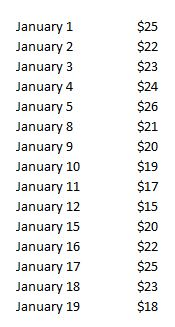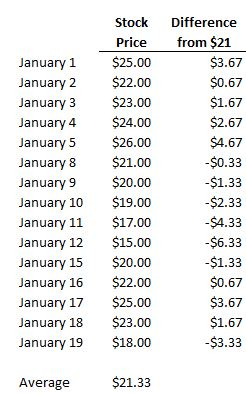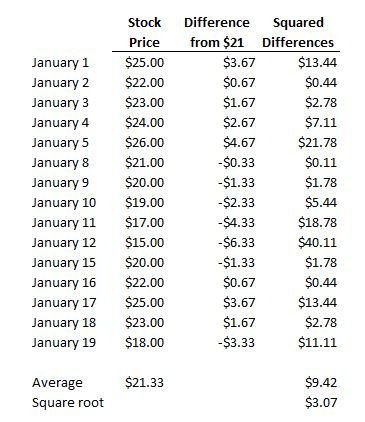# Variance

Updated July 11, 2021

## What is Variance?

Variance is a statistical measure of how much a set of observations differ from each other.

In accounting and financial analysis, variance also refers to how much an actual expense deviates from the budgeted or forecast amount.

## How Does Variance Work?

For example, let's say Company XYZ stock has the following prices:The average of these prices is \$21.33. To calculate the variance, we see how "far away" each day's stock price is from \$21, like this:Notice that some of the differences are negative. Because we're going to calculate the average difference, the negative numbers create a mathematical problem (they'll offset the positive numbers and screw up the calculation). To avoid this, we square each difference so that each difference is positive, like this:The last step is simply calculating the average of those squared differences, which is \$9.42, and then taking the square root of that number to get the amount by which Company XYZ stock tends to vary from its average price.

The square root is \$3.07, meaning that when Company XYZ deviates from that \$21 average, it tends to do so by about \$3.07.

## Why Does Variance Matter?

Variance is a measure of volatility because it measures how much a stock tends to deviate from its mean. The higher the variance, the more wildly the stock fluctuates. Accordingly, the higher the variance, the riskier the stock.

All of our content is verified for accuracy by Paul Tracy and our team of certified financial experts. We pride ourselves on quality, research, and transparency, and we value your feedback. Below you'll find answers to some of the most common reader questions about Variance.
##### Be the first to ask a question

Paul Tracy - 140Paul has been a respected figure in the financial markets for more than two decades. Prior to starting InvestingAnswers, Paul founded and managed one of the most influential investment research firms in America, with more than 2 million monthly readers.